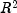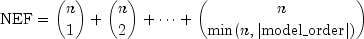JMSLTM Numerical Library 7.2.0
com.imsl.stat

Class ANOVAFactorial

• All Implemented Interfaces:
Serializable, Cloneable

public class ANOVAFactorial
extends Object
implements Serializable, Cloneable
Analyzes a balanced factorial design with fixed effects.

Class ANOVAFactorial performs an analysis for an n-way classification design with balanced data. For balanced data, there must be an equal number of responses in each cell of the n-way layout. The effects are assumed to be fixed effects. The model is an extension of the two-way model to include n factors. The interactions (two-way, three-way, up to n-way) can be included in the model, or some of the higher-way interactions can be pooled into error. setModelOrder specifies the number of factors to be included in the highest-way interaction. For example, if three-way and higher-way interactions are to be pooled into error, specify modelOrder = 2. (By default, modelOrder = nSubscripts - 1 with the last subscript being the error subscript.) PURE_ERROR indicates there are repeated responses within the n-way cell; POOL_INTERACTIONS indicates otherwise.

Class ANOVAFactorial requires the responses as input into a single vector y in lexicographical order, so that the response subscript associated with the first factor varies least rapidly, followed by the subscript associated with the second factor, and so forth. Hemmerle (1967, Chapter 5) discusses the computational method.

Example 1, Example 2, Example 3, Serialized Form
• Field Summary

Fields
Modifier and Type Field and Description
static int POOL_INTERACTIONS
Indicates factor nSubscripts is not error.
static int PURE_ERROR
Indicates factor nSubscripts is error.
• Constructor Summary

Constructors
Constructor and Description
ANOVAFactorial(int nSubscripts, int[] nLevels, double[] y)
Constructor for ANOVAFactorial.
• Field Detail

• POOL_INTERACTIONS

public static final int POOL_INTERACTIONS
Indicates factor nSubscripts is not error.
Constant Field Values
• PURE_ERROR

public static final int PURE_ERROR
Indicates factor nSubscripts is error.
Constant Field Values
• Constructor Detail

• ANOVAFactorial

public ANOVAFactorial(int nSubscripts,
int[] nLevels,
double[] y)
Constructor for ANOVAFactorial.
Parameters:
nSubscripts - an int scalar containing the number of subscripts. Number of factors in the model + 1 (for the error term).
nLevels - an int array of length nSubscripts containing the number of levels for each of the factors for the first nSubscripts-1 elements. nLevels[nSubscripts-1] is the number of observations per cell.
y - a double array of length nLevels * nLevels * ... * nLevels[nSubscripts-1] containing the responses. y must not contain NaN for any of its elements, i.e., missing values are not allowed.
Throws:
IllegalArgumentException - is thrown if nLevels.length, and y.length are not consistent
• Method Detail

• compute

public final double compute()
Analyzes a balanced factorial design with fixed effects.
Returns:
a double scalar containing the p-value for the overall F test
• getANOVATable

public double[] getANOVATable()
Returns the analysis of variance table. Note that the compute method must be invoked first before invoking this method. Otherwise, the method throws a NullPointerException exception.
Returns:
a double array containing the analysis of variance table. The analysis of variance statistics are given as follows:  Element Analysis of Variance Statistics 0 degrees of freedom for the model 1 degrees of freedom for error 2 total (corrected) degrees of freedom 3 sum of squares for the model 4 sum of squares for error 5 total (corrected) sum of squares 6 model mean square 7 error mean square 8 overall F-statistic 9 p-value 10(in percent) 11 adjusted(in percent) 12 estimate of the standard deviation 13 overall mean of y 14 coefficient of variation (in percent)

• getMeans

public double[] getMeans()
Returns the subgroup means. Note that the compute method must be invoked first before invoking this method. Otherwise, the method throws a NullPointerException exception.
Returns:
a double array containing the subgroup means
• getTestEffects

public double[][] getTestEffects()
Returns statistics relating to the sums of squares for the effects in the model. Note that the compute method must be invoked first before invoking this method. Otherwise, the method throws a NullPointerException exception.
Returns:
a double matrix containing statistics relating to the sums of squares for the effects in the model. Here,where n is given by nSubscripts if ANOVAFactorial.POOL_INTERACTIONS is specified; otherwise, nSubscripts - 1. Suppose the factors are A, B, C, and error. With modelOrder = 3, rows 0 through NEF-1 would correspond to A, B, C, AB, AC, BC, and ABC, respectively.

The columns of the output matrix are as follows:

 Column Description 0 degrees of freedom 1 sum of squares 2 F-statistic 3 p-value

• setErrorIncludeType

public void setErrorIncludeType(int type)
Sets error included type.
Parameters:
type - an int scalar. ANOVAFactorial.PURE_ERROR, the default option, indicates factor nSubscripts is error. Its main effect and all its interaction effects are pooled into the error with the other (modelOrder + 1)-way and higher-way interactions. ANOVAFactorial.POOL_INTERACTIONS indicates factor nSubscripts is not error. Only (modelOrder + 1)-way and higher-way interactions are included in the error.
• setModelOrder

public void setModelOrder(int modelOrder)
Sets the number of factors to be included in the highest-way interaction in the model.
Parameters:
modelOrder - an int scalar containing the number of factors to be included in the highest-way interaction in the model. modelOrder must be in the interval [1, nSubscripts - 1 ]. For example, a modelOrder of 1 indicates that a main effect model will be analyzed, and a modelOrder of 2 indicates that two-way interactions will be included in the model. Default: modelOrder = nSubscripts - 1
JMSLTM Numerical Library 7.2.0